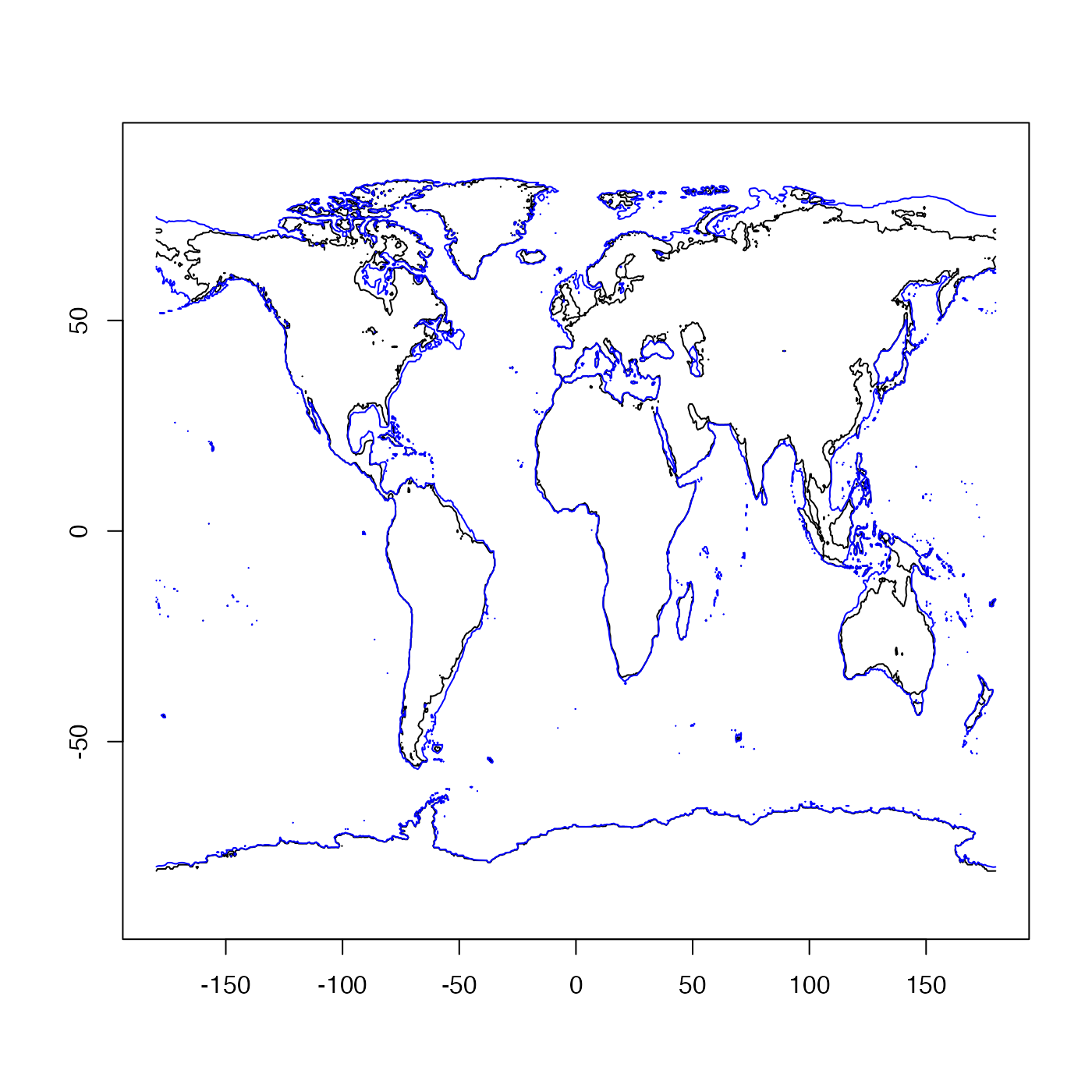This provides something analogous to contour(), but with the ability to flip x and y. Setting revy=TRUE can be helpful if the y data represent pressure or depth below the surface.

oce.contour(
x,
y,
z,
revx = FALSE,
revy = FALSE,
tformat,
drawTimeRange = getOption("oceDrawTimeRange"),
debug = getOption("oceDebug"),
...
)

## Arguments

x

values for x grid.

y

values for y grid.

z

matrix for values to be contoured. The first dimension of z must equal the number of items in x, etc.

revx

set to TRUE to reverse the order in which the labels on the x axis are drawn

revy

set to TRUE to reverse the order in which the labels on the y axis are drawn

logical value indicating whether the contours should be added to a pre-existing plot.

tformat

time format; if not supplied, a reasonable choice will be made by oce.axis.POSIXct(), which draws time axes.

drawTimeRange

logical, only used if the x axis is a time. If TRUE, then an indication of the time range of the data (not the axis) is indicated at the top-left margin of the graph. This is useful because the labels on time axes only indicate hours if the range is less than a day, etc.

debug

a flag that turns on debugging; set to 1 to information about the processing.

...

optional arguments passed to plotting functions.

Dan Kelley

## Examples

library(oce)
data(topoWorld)
# coastline now, and in last glacial maximum
lon <- topoWorld[["longitude"]]
lat <- topoWorld[["latitude"]]
z <- topoWorld[["z"]]
oce.contour(lon, lat, z, levels = 0, drawlabels = FALSE)
oce.contour(lon, lat, z, levels = -130, drawlabels = FALSE, col = "blue", add = TRUE)